# 剑指offer(11-25题)详解

剑指offer系列## 11 二进制种1的个数★

1<<0=1 =00000000000000000000000000000001
1<<1=2 =00000000000000000000000000000010
1<<2=4 =00000000000000000000000000000100
1<<3=8 =00000000000000000000000000001000
. . . . . .
1<<30=230 =01000000000000000000000000000000
1<<31=-231 =10000000000000000000000000000000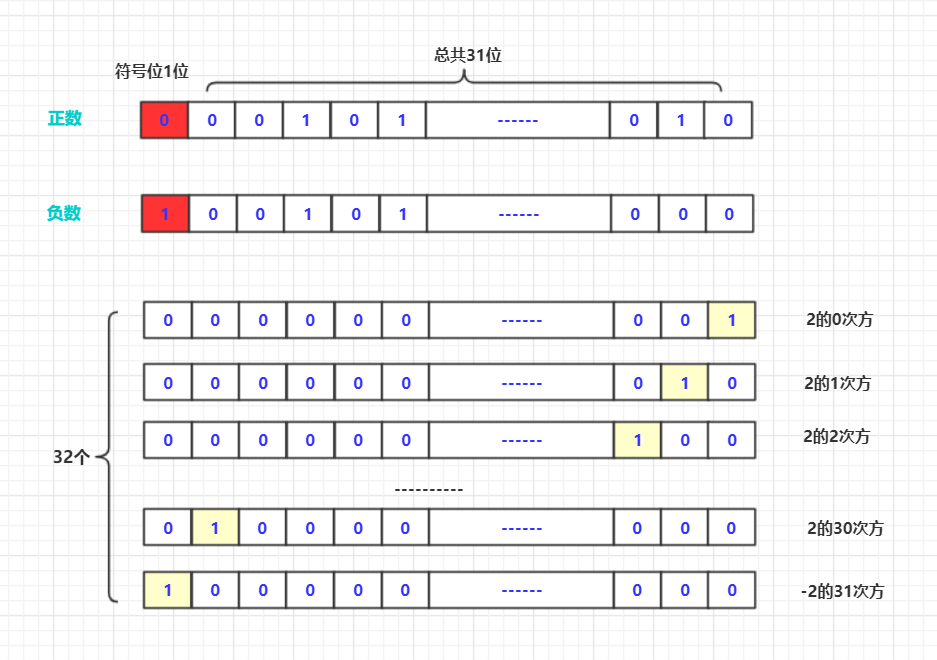public int NumberOf1(int n) {
int va=0;
String num=Integer.toBinaryString(n);
for(int i=0;i<num.length();i++)
{
if(num.charAt(i)=='1')
{
va++;
}
}
return va;
}
public int NumberOf2(int n) {
int va=0;
for(int i=0;i<32;i++)
{
if((n&(1<<i))!=0)
{
va++;
}
}
return va;
}
public int NumberOf3(int n) {//上面的差不多，可能慢一点
int va=0;
int num=1;
for(int i=0;i<32;i++)
{
if((n&num)!=0)
{
va++;
}
num*=2;
}
return va;
}


评论区参考：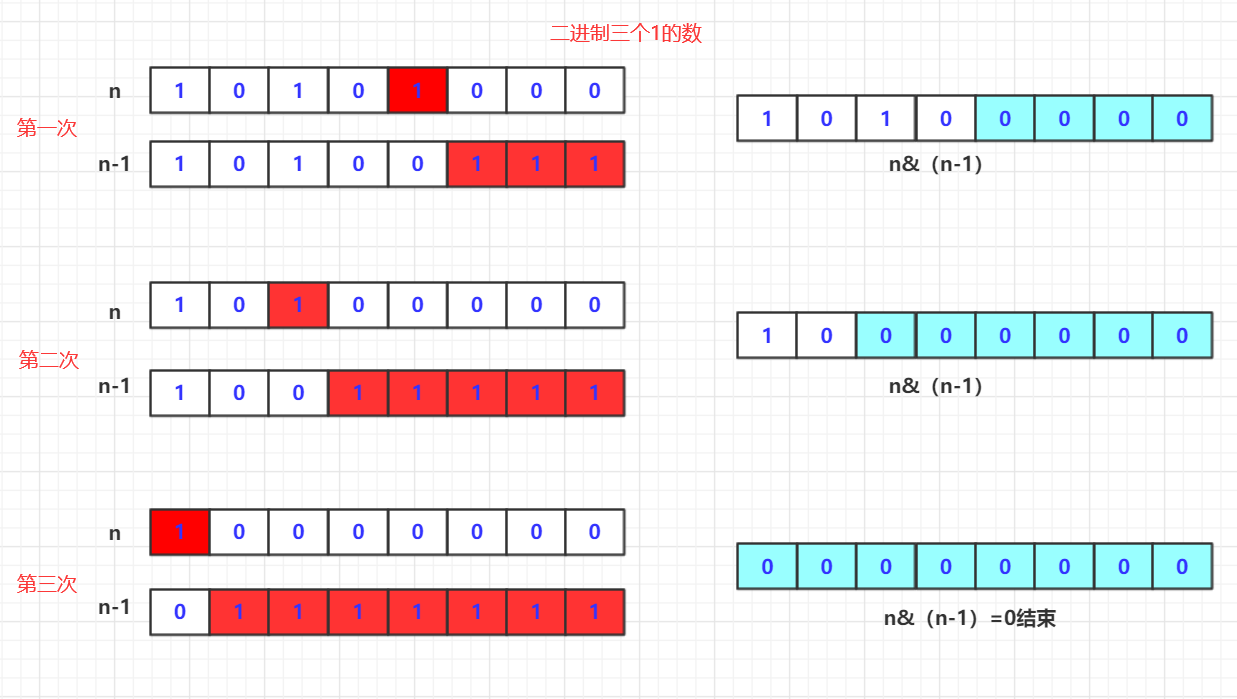public class Solution {
public int NumberOf1(int n) {
int count=0;
while (n!=0) {
n=n&(n-1);
count++;
}
return count;
}
}


## 12 数值的正数次方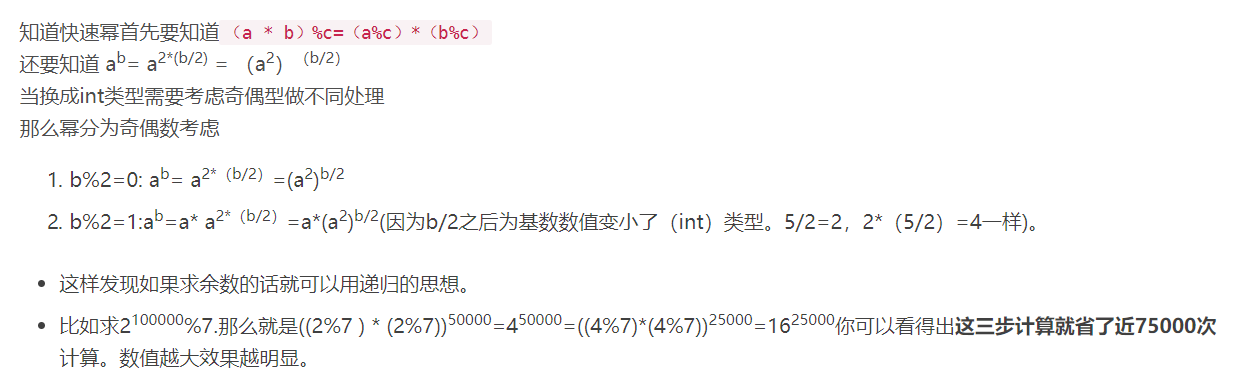public double Power(double base, int exponent) {
return Math.pow(base, exponent);
}
public double Power(double base, int exponent) {
if(base==0)return 0;
if(exponent<0) {exponent=-exponent;base=1/base;}
if(exponent==0)return 1;
else if (exponent % 2 == 0) {
return Power(base*base, exponent/ 2) ;
} else
return base*Power(base*base, (exponent-1)/ 2);
}


## 13 调整数组顺序使奇数位于偶数前面

• 需要新开数组team复制(克隆)array数组
• 进行两次遍历team，一次从左向右找奇数从左向右赋值给array对应位置。
• 一次从右向左遍历team将数值从右往左赋值给array对应位置。
public class Solution {
public void reOrderArray(int [] array) {
int team[]=array.clone();
int index=0;
for(int i=0;i<team.length;i++)
{
if((team[i]&1)==1)//如果为奇数
array[index++]=team[i];
}
index=team.length-1;
for(int i=team.length-1;i>=0;i--)
{
if((team[i]&1)==0)
array[index--]=team[i];
}
}
}


参考讨论区：

## 14 链表中倒数第K个节点

/*
public class ListNode {
int val;
ListNode next = null;

ListNode(int val) {
this.val = val;
}
}*/
public class Solution {
public ListNode FindKthToTail(ListNode head, int k) {

int count = 0;
while (team != null) {
count++;
team = team.next;
}
int value = count - k;
int index = 0;
if (index == value) {
}
index++;

}
}
}



参考讨论区

比如递归先到头，返回的时候对整型数值叠加符合题意返回，判断处理即可！
还有就是定义快慢指针。这个思想就像两辆车中间拖个绳子，前车走的如果超过绳子长度就拉着后车保持绳子长度跑。如果绳子没拉直那就肯定不符合题意哒！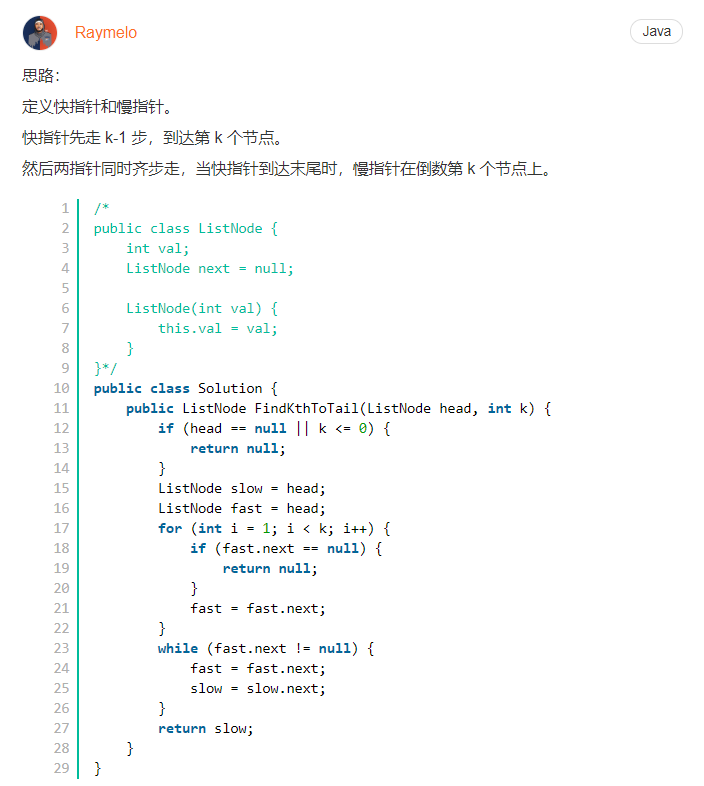## 15 反转链表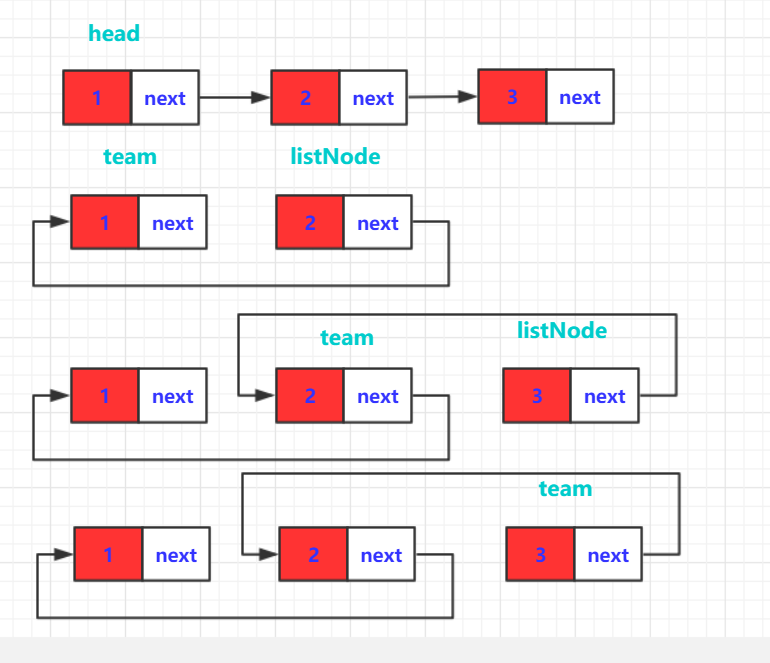public  static class ListNode {
int val;
ListNode next = null;

ListNode(int val) {
this.val = val;
}
}

listNode.next = team;// 将它指向lsitNode
team = listNode;// team指向listnode
}
}
return team;
}


public static ListNode ReverseList(ListNode head) {
else {
return node;
}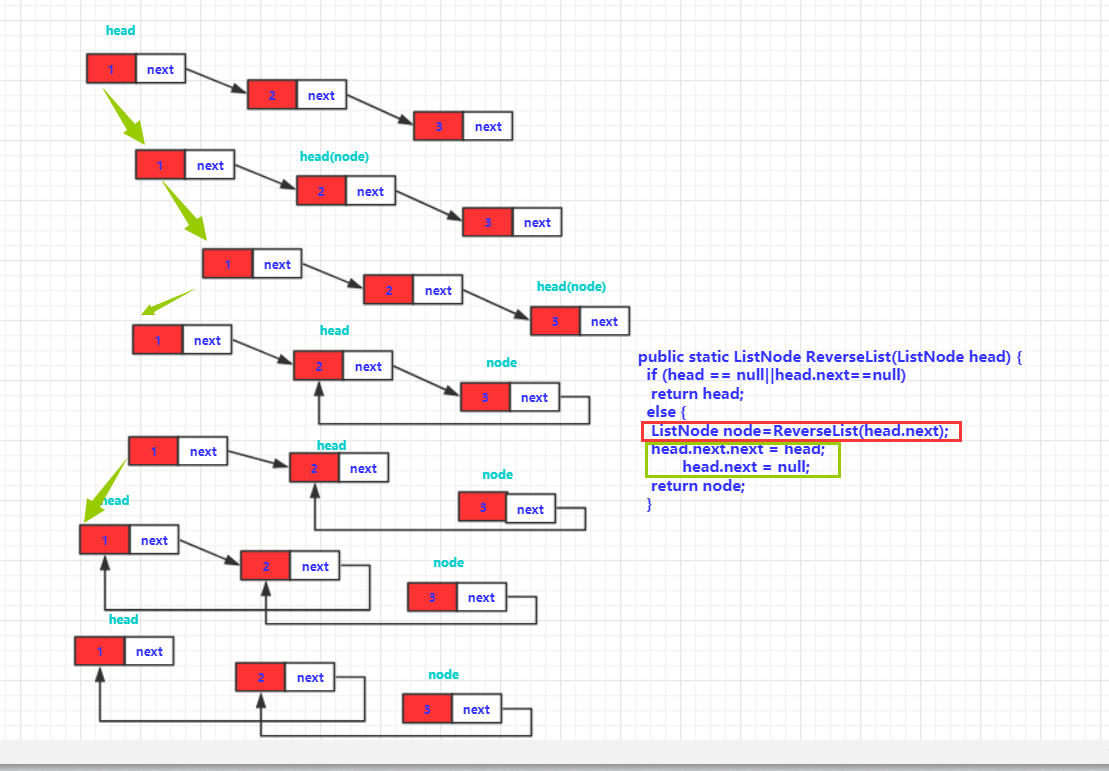## 16 合并两个排序的链表

/*
public class ListNode {
int val;
ListNode next = null;

ListNode(int val) {
this.val = val;
}
}*/
public class Solution {
public ListNode Merge(ListNode list1,ListNode list2) {
if(list1==null)return list2;
else if (list2== null) {return list1;

}
ListNode value=new ListNode(0);
ListNode team=value;
while(list1!=null&&list2!=null)
{
if(list1.val<list2.val)
{
team.next=new ListNode(list1.val);
list1=list1.next;
team=team.next;
if(list1==null)
{
team.next=list2;
break;
}
}
else {
team.next=new ListNode(list2.val);
list2=list2.next;
team=team.next;
if(list2==null)
{
team.next=list1;
break;
}
}
}
value=value.next;
return value;
}
}


参考讨论区：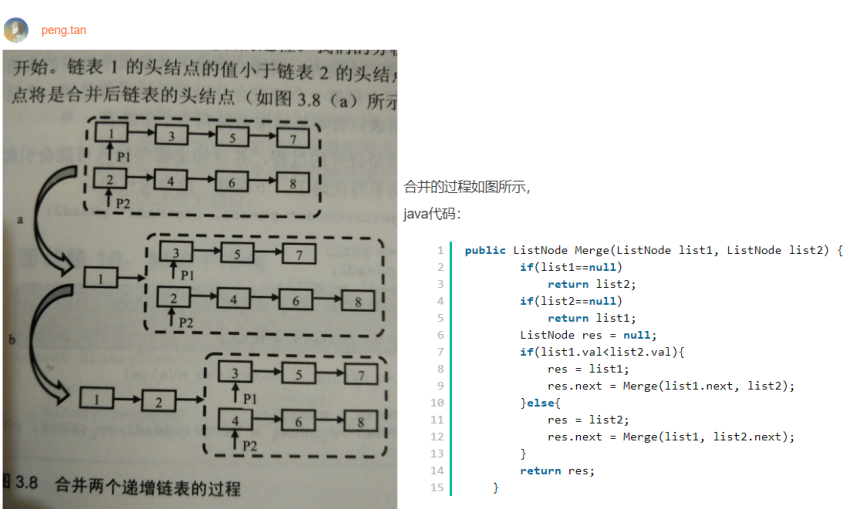## 17 树的子结构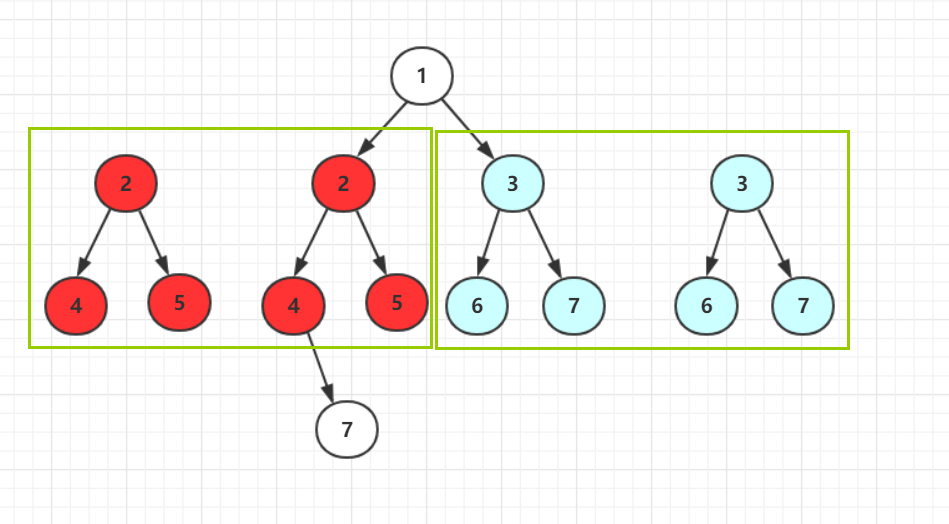/**
public class TreeNode {
int val = 0;
TreeNode left = null;
TreeNode right = null;

public TreeNode(int val) {
this.val = val;

}

}
*/
import java.util.ArrayDeque;
import java.util.Queue;
public class Solution {
public boolean HasSubtree(TreeNode root1,TreeNode root2) {

Queue<TreeNode>q1=new ArrayDeque<TreeNode>();
if (root2==null||root1==null)
return false;
else {
while (!q1.isEmpty()) {
TreeNode t1=q1.poll();
if(jud(t1, root2))
return true;
else {
}
}
return false;
}
}
public boolean jud(TreeNode root1,TreeNode root2) {

if(root2==null)return true;
else if(root1==null)
{
return false;
}
else if(root1.val==root2.val) {
return (jud(root1.left, root2.left)&&jud(root1.right,root2.right));
}
else {
return false;
}
}
}


参考评论区：

## 18 二叉树的镜像

二叉树的镜像定义：源二叉树
8
/  \
6   10
/ \  / \
5  7 9 11
镜像二叉树
8
/  \
10   6
/ \  / \
11 9 7  5


二叉树的镜像定义：源二叉树
8
/  \
6   10
/ \  / \
5  7 9  11
//step1 第三层交换
8
/  \
6   10
/ \  / \
7  5 11  9
//step2  第二层交换，第一层不需要交换
8
/  \
10   6
/ \  / \
11 9 7   5


/**
public class TreeNode {
int val = 0;
TreeNode left = null;
TreeNode right = null;
public TreeNode(int val) {
this.val = val;
}
}
*/
public class Solution {
public void Mirror(TreeNode root) {
if(root==null) return;
TreeNode teamNode=root.right;
root.right=root.left;
root.left=teamNode;
if(root.left!=null) {Mirror(root.left);}
if(root.right!=null) {Mirror(root.right);}
}
}


## 19 顺时针打印矩阵

• 大家都知道是顺时针进行旋转式的输出。那么这本身就是一次运动，那么我们就可以模拟这个光标的运动，一直到结束。
• 想法虽然简单，但是实现起来很麻烦！比如，你可能需要用参数标记当前的方向，还可能需要用个boolean数组判断是否走过和是否越界问题。还可能需要多个if else当判定当前结束选择下一个方向。想想这个代码一定是很臃肿的。虽然可以实现，有兴趣的可以尝试。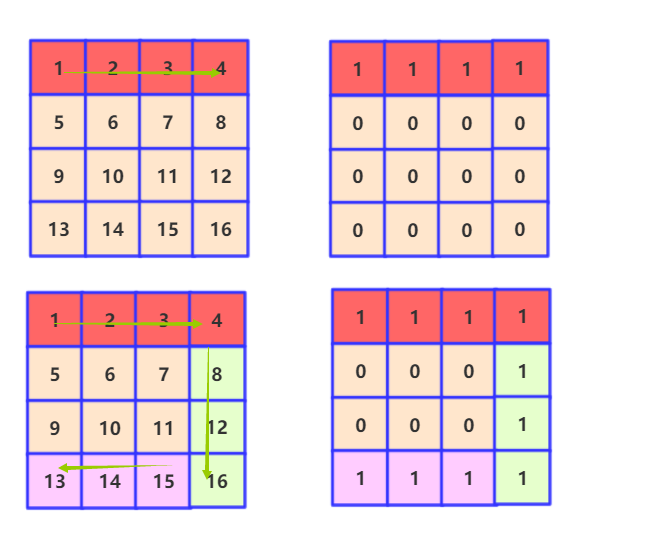• 先不考虑走完的问题。每次走的四次开始的坐标和结束的坐标是可以算出来的！因为相当于每走一圈横纵坐标都减二。而开始和结束位置分别加1和减1。所以每次走的结束位置可以准确表示出来。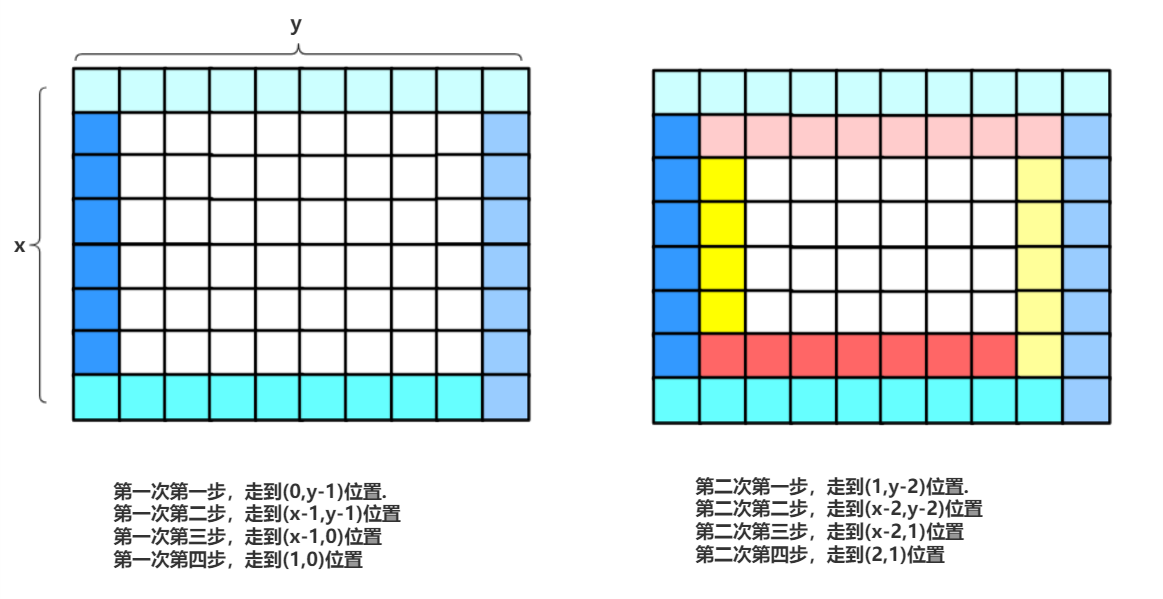• 考虑走完的问题。肯定是根据窄的走完。问题还有奇偶的问题。如果偶数的话可以刚好走完，而奇数需要特殊判断一下省略最后一次循环一些步骤才行！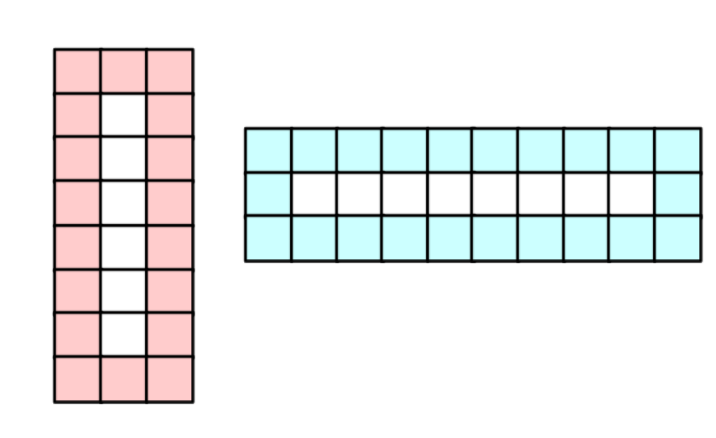• 其他注意点。当循环添加一轮的时候，实际上当前的坐标是有变化的。需要手动修正一下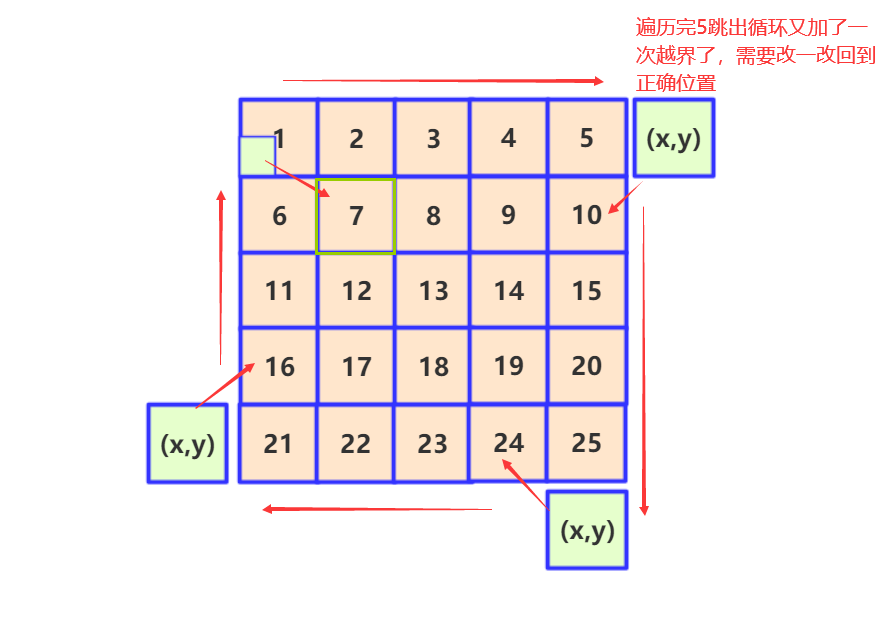import java.util.ArrayList;
public class Solution {
public ArrayList<Integer> printMatrix(int [][] matrix) {
ArrayList<Integer> list=new ArrayList<Integer>();
int xlen=matrix.length;
int ylen=matrix.length;
int min=Math.min(xlen, ylen);
int x=0;//上下 纵坐标
int y=0;//左右 横坐标
for(int i=0;i<(min+1)/2;i++)
{
if(i==xlen/2)continue;
if(i==ylen/2)continue;
}
return list;
}
}


## 20 包含main函数的栈

1. push加入的时候是不是更小，如果更小的话需要更新这个min。
2. pop元素是不是和min一样大。如果比min大不需要管。但是如果和min一样大那么就需要遍历重新确定min。当然整个函数的最大开销也就在这里了。

import java.util.Stack;
public class Solution {
private int a[]=new int ;
private int index=0 ;
private int min=Integer.MAX_VALUE;
public void push(int node) {
a[index]=node;
if(a[index]<min)min=a[index];
index++;
}
public void pop() {
if(a[--index]==min)
{
min=Integer.MAX_VALUE;
for(int i=0;i<index;i++)
{
if(a[i]<min)
{
min=a[i];
}
}
}
}
public int top() {
return a[index-1];
}
public int min() {
return min;
}
}


## 21 栈的压入、弹出序列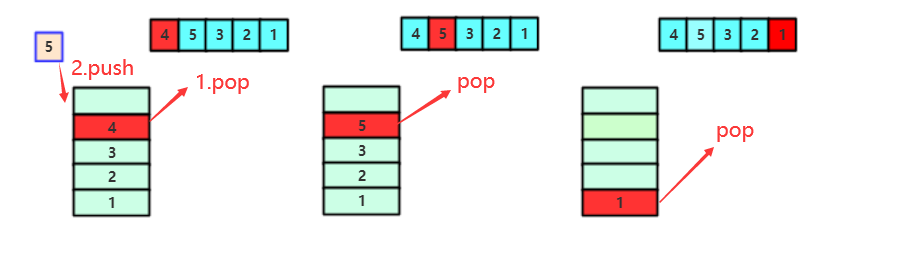import java.util.ArrayList;
import java.util.Stack;
public class Solution {
public boolean IsPopOrder(int[] pushA, int[] popA) {
Stack<Integer> stack = new Stack<Integer>();
int index = 0;
for (int i = 0; i < pushA.length; i++) {// 找到第一个push元素的位置
stack.push(pushA[i]);
//while (!stack.isEmpty()) {
while (!stack.isEmpty()&&stack.peek() == popA[index]) {
stack.pop();
index++;
//}
//break;
}
}
if (stack.size() == 0)
return true;
else {
return false;
}
}
}


## 22 从下往上打印二叉树

import java.util.ArrayDeque;
import java.util.ArrayList;
import java.util.Queue;
/**
public class TreeNode {
int val = 0;
TreeNode left = null;
TreeNode right = null;
public TreeNode(int val) {
this.val = val;
}
}
*/
public class Solution {
public ArrayList<Integer> PrintFromTopToBottom(TreeNode root) {

ArrayList<Integer>list=new ArrayList<Integer>();
if(root==null)return list;
Queue<TreeNode>q1=new ArrayDeque<>();
while (!q1.isEmpty()) {
TreeNode node=q1.poll();
}
return list;
}
}


## 23 二叉搜索树的后序遍历序列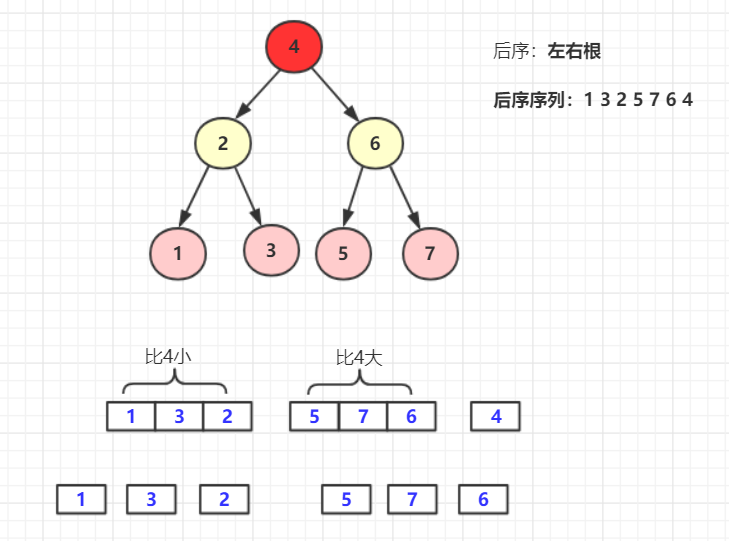public class Solution {
public boolean VerifySquenceOfBST(int [] sequence) {
if (sequence.length == 0)
return false;
return jud(sequence, 0, sequence.length - 1);
}
public boolean jud(int a[],int left,int right)//左右包含左和右
{
int index=0;int mid=0;
if(left>=right)return true;
for(;index<right;index++)
{
if(a[index]>a[right])
break;
}
mid=index;
for(;index<right;index++)
{
if(a[index]<a[right])break;
}
if(index!=right)return false;
else {
return jud(a, left, mid-1)&&jud(a, mid, right-1);
}
}
}


## 24 二叉树中和为某一值的路径

dfs深度优先遍历。要注意的是要遍历到叶子节点的路径。如果有中途遍历的时候路径满足而非叶子节点是不停止的。

dfs(TreeNode root, int target, ArrayList<Integer> list, ArrayList<ArrayList<Integer>> list2


import java.util.ArrayList;

import java.util.Comparator;
/**
public class TreeNode {
int val = 0;
TreeNode left = null;
TreeNode right = null;

public TreeNode(int val) {
this.val = val;
}
}
*/
public class Solution {
public  ArrayList<ArrayList<Integer>> FindPath(TreeNode root, int target) {

ArrayList<Integer> team = new ArrayList<Integer>();
ArrayList<ArrayList<Integer>> list = new ArrayList<ArrayList<Integer>>();
if (root == null) {
return list;
}
dfs(root, target, team, list);
return list;
}

static Comparator<ArrayList<Integer>> comparator = new Comparator<ArrayList<Integer>>() {

public int compare(ArrayList<Integer> o1, ArrayList<Integer> o2) {
// TODO Auto-generated method stub
return o2.size() - o1.size();
}
};

public  void dfs(TreeNode root, int target, ArrayList<Integer> list, ArrayList<ArrayList<Integer>> list2) {
if (target < 0) {// 停止
} else {
target -= root.val;
if (root.left != null)
dfs(root.left, target, list, list2);

if (root.right != null)
dfs(root.right, target , list, list2);
if (root.left==null&&root.right==null&&target == 0) {//叶子节点
ArrayList<Integer> list3 = new ArrayList<Integer>();
}
target+=root.val;
list.remove((Integer) root.val);
}
}
}


参考评论区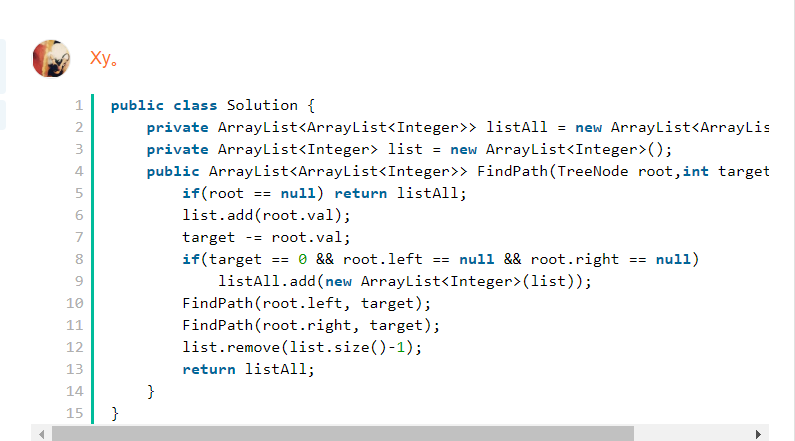## 25 复杂链表的复制

1. 它每个节点有一个next，有一个random。也就是一个指向后面，一个瞎指的。 如果两个指针同时考虑真的太复杂了，那我先分开考虑。只看next他就是一个链表。对吧(先不考虑循环)
2. 我们用个RandomListNode list[]遍历链表将链表各个节点存进去。RandomListNode clone[]是我们想克隆的数组。至于克隆数组每个对应位子应该和list是相似的。并且每个next应该指向下一位clone[i].next=clone[i+1],至于每个random的位置。我们可以挨个遍历list中random的相对位置，然后clone的random也指向相应位置。这个是开销最大的部分，时间复杂度为O(n2).但是我自己没想到更好的方法，后面参考题解如果有更好方法会进行更新吧。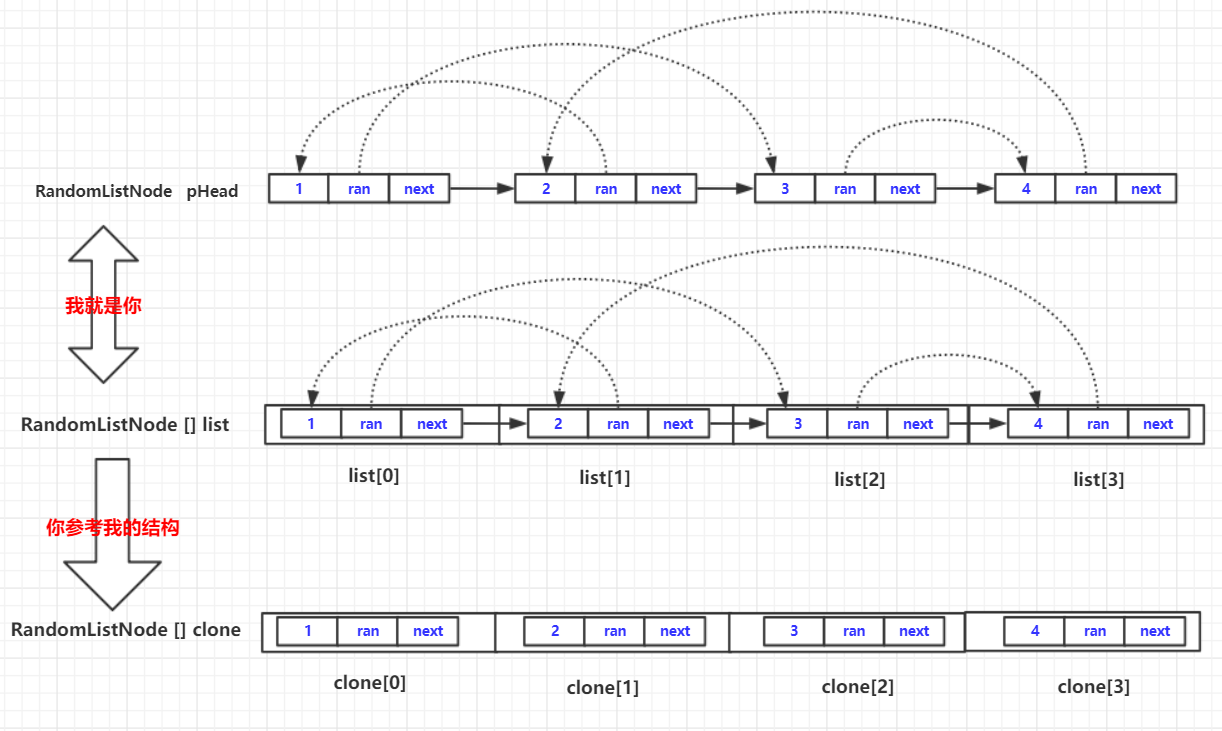实现代码为：
/*
public class RandomListNode {
int label;
RandomListNode next = null;
RandomListNode random = null;

RandomListNode(int label) {
this.label = label;
}
}
*/
public class Solution {
//step1 计算有多少个
int count=0;
while(team!=null)
//step2 将每个位置的指针存在
RandomListNode list[]=new RandomListNode[count+1];
RandomListNode clone[]=new RandomListNode[count+1];
count=0;
while(team!=null)
{
clone[count]=new RandomListNode(team.label);
list[count++]=team;
team=team.next;
}
//step3 克隆指针

for(int i=0;i<count;i++)
{
clone[i].next=clone[i+1];
for(int j=0;j<count;j++)
{
if(list[i].random==list[j])
{
clone[i].random=clone[j];
break;
}
}
}

return clone;
}
}


参考讨论区：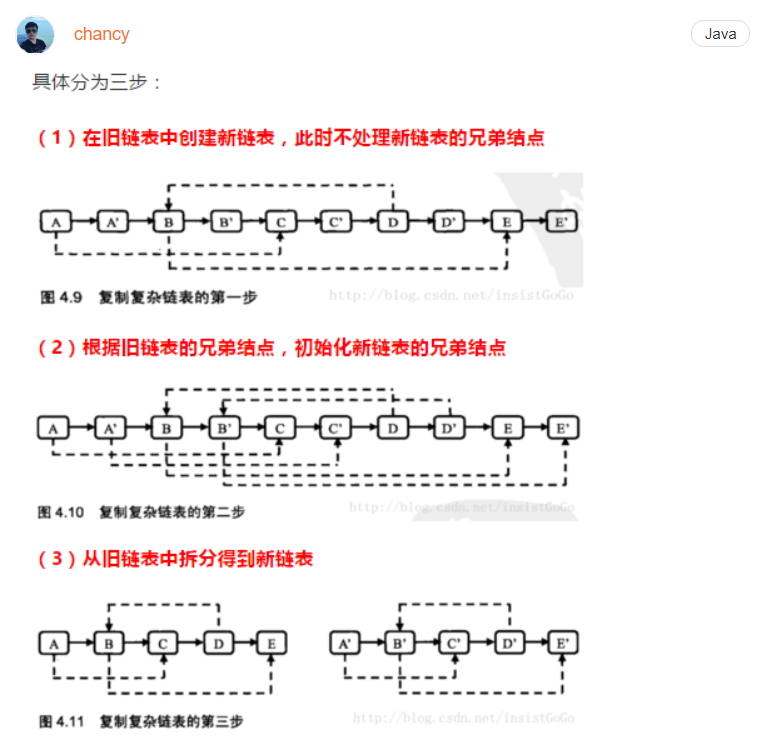链接：https://www.nowcoder.com/questionTerminal/f836b2c43afc4b35ad6adc41ec941dba?f=discussion

/*
*解题思路：
*1、遍历链表，复制每个结点，如复制结点A得到A1，将结点A1插到结点A后面；
*2、重新遍历链表，复制老结点的随机指针给新结点，如A1.random = A.random.next;
*3、拆分链表，将链表拆分为原链表和复制后的链表
*/
public class Solution {
return null;
}

//1、复制每个结点，如复制结点A得到A1，将结点A1插到结点A后面；
while(currentNode != null){
RandomListNode cloneNode = new RandomListNode(currentNode.label);
RandomListNode nextNode = currentNode.next;
currentNode.next = cloneNode;
cloneNode.next = nextNode;
currentNode = nextNode;
}

//2、重新遍历链表，复制老结点的随机指针给新结点，如A1.random = A.random.next;
while(currentNode != null) {
currentNode.next.random = currentNode.random==null?null:currentNode.random.next;
currentNode = currentNode.next.next;
}

//3、拆分链表，将链表拆分为原链表和复制后的链表
while(currentNode != null) {
RandomListNode cloneNode = currentNode.next;
currentNode.next = cloneNode.next;
cloneNode.next = cloneNode.next==null?null:cloneNode.next.next;
currentNode = currentNode.next;
}

}
}©️2019 CSDN 皮肤主题: 代码科技 设计师: Amelia_0503